Point Reflection in Transformational Geometry

Let A be a given point in the plane, and let P be another point in the plane. We construct the point P’ the point reflection of P with center A, as follows: let P’ be the point on line AP distinct from P with |AP’| = |AP|. (Special case: if P=A, then define P’= A also.) In other words, A is the midpoint of PP'.

This defines a function or transformation H_A that takes P to P’. This transformation is also sometimes called point reflection or point symmetry. This transformation is the same as a half-turn, a rotation by 180 degrees.

1.1 Point Reflection Images using Sketchpad

In this first Sketchpad exercise we explore several important properties of point reflections and then solve a problem using these properties. The properties are:

• Point reflections are isometries (i.e., point reflections preserve distance).
• A point reflection maps a line m to a parallel line m'.

Experiment 1A. Image of 2 points.

• In a new sketch, draw 3 points A, B, C with the point tool. Then mark A as a center.
• Rotate B and C by 180 degrees with center A. Now construct segments to form triangle ABC and triangle AB’C’.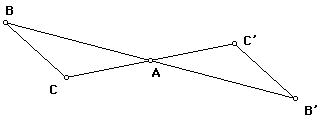• Drag points B and C at will and observe how the distances |BC| and |B’C’| are related. (You can measure them if you like.) How could you use triangle congruence to prove this relationship?
• Also, observe that segment BC is always parallel to segment B’C’. How can this be proved?
• If a point D is on line BC, explain why the image D' of D is on line B'C'. This means that the image of line BC is line B'C'.

Experiment 1B. Image of lines, circles, etc.

Suppose S is a set of points in the plane, and F is a transformation of the plane. The F-image S’ of set S (also written as F(S)) is the set of points P’ for which P’ = F(P) for a point P in S. Working with the image of a set rather than a point at a time is a very powerful way to understand and use a transformation.

In this experiment we observe that when a point moves along a geometrical object, how its images moves along the image of the object.

• Continuing with the figure from 1A, draw a point D and construct D', the point reflection of D in A.
• Now select D along with segment BC (CAREFUL: Just select the segment, not B or C.) Then choose the menu item Edit>Action Button>Animation setting the slow speed. Now by double-clicking the button, D moves on one segment and D' moves along its image.

What happens if you animate D along the segment B'C' instead of BC?

• Now try another image. Draw a circle c and rotate the circle by 180 degrees about A to form the point reflection image c' of the original circle. Now animate the same D on c and see how D' moves along c'.

A figure F has A as a center of Point Symmetry if the half-turn H_A is a symmetry of F. This means that the H_A image of F is F itself. Consider and explain these examples:

• For a segment GH, the midpoint M of GH is a center of point symmetry of the segment. Does the segment have another center of point symmetry?
• For a LINE, what are the centers of point symmetry?
• In the figure of 1A, explain why the quadrilateral BCB'C' is a parallelogram with center of point symmetry A.
• Let F be a figure consisting of two parallel lines and a transversal, specifically, a line AB and two parallel lines a, through A, and b, through B. Then the midpoint M of AB is a center of point symmetry of this figure.

1.2 Solving a Problem with a Point reflection

Here is a curious geometry problem.

Version 1. Given two lines m and n and a point A, find a point M on m and a point N on n so that A is the midpoint of MN.

It may not be clear how to attack this problem, but point reflections give an approach to the problem. It can be reformulated thus:

Version 2. Given two lines m and n and a point A, find a point M on m and a point N on n so that N is the point reflection of M with center A.

Finally, there is a third version:

Version 3. Given two lines m and n and a point A, find a point M on m and on the image of n by the point reflection with center A.

Experiment 1C.

• Create a sketch with point A and two lines, m = line BC and n = line DE.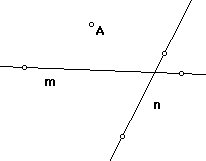• Construct the image n' of line n by the point reflection with center A.
• Define M to be the intersection point of m and n'; define N as the point reflection of M.
• Check that this really solves the problem. Think through why this solution works. What are the special cases that have no solution?
• Solve a new problem. Draw a sketch with a point A and two circles c and d. The problem is to find all points P on c and Q on d so that A is the midpoint of PQ. Carry out a solution with Sketchpad, inspired by the previous problem. What are the possible numbers of solutions PQ?

2. Composition of Point reflections

One of the powerful concepts about transformations is the concept of composition. We can get some interesting geometric figures by composing point reflections.

Experiment 2A.

1. In a new sketch, draw two points A and B and then draw a shape S as a polygon interior. Any irregular shape will do.
2.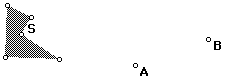3. Now rotate S by 180 degrees with center A to get S'. Continue rotating by 180 degrees as follows: rotate S' with center B to get S''. S'' is obtained from S by the composition H_B H_A. From your observation what kind of transformation relates S and S''?
4. Continue adding to this figure with 180-degree rotations. Next rotate S'' with center A to get S'''; then rotate S''' with center B to get S'''.
5. Now start again with S and rotate with center B first, then A, for several steps.
6. Observe the pattern you get as you drag S or as you drag A or B. In particular, observe that some shapes appear to be translations of each other and some not. How are the shapes spaced? Is there any consistency as you drag S?

Experiment 2B

This experiment explains composition of point reflections in terms of elementary geometry.

This figure would usually be constructed from a triangle and its midpoints, but instead, construct the figure in a special way.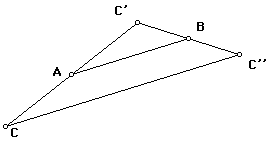1. Rotate C by 180 degrees with center A to get C'. Then in the same way, take the point reflection of C' with center B. Connect the points as in the figure.
2. Measure the lengths of AB and CC''.
3. Explain why segment CC'' is parallel to segment AB, in the same direction and twice as long wherever you move C.
4. Add a shape S to the figure along with S', the point reflection of S with center A, and S'', the point reflection of S' with center B. Experiment by moving point C to various vertices of S. Notice how the corresponding points of the other shapes coincide with C' and C''.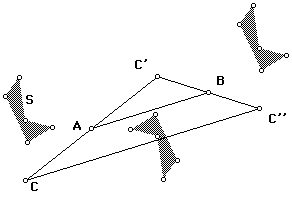If H_A is the point reflection with center A and H_B is the point reflection with center B, then C'' = H_B(C') = H_B(H_A(C)). If we ignore the intermediate step and move straight from C to C'', we can think of this as a single transformation. This transformation T is the composition of the two point reflections. We denote this as a product. This T = H_B H_A, and T(C) = C''.

Experiment 2C. Translations as compositions

Sketchpad can create transformations that are compositions.

1. Continue with the previous sketch. Hide the point C' so that only C and C'' are showing. Select these two points. Now choose Define Transform from the Transform menu and name the transform T2pt
2. Now hide S'. Then select S'' and choose T from the transform menu. Do this several times and observe the pattern.
3. Now define a new transform U = H_A H_B. Do this by drawing and new point P and taking first the point reflection with center B and then the point reflection with center A. Then hide P' and again Define Transform.
4. What is this transformation?
5. Now lets do an experiment with line reflections. Make a new irregular shape X with the same number of vertices as S. Also, construct the lines a and b through A and B that are perpendicular to segment AB.
6. Reflect X across a to get X' and then reflect X' across b to get X''. Move X so that its vertices coincide with those of S and compare how X'' and S'' are related. Also, tell how X' and S' are related.
7. Hide X', select X and X'' and choose Define Transform again. Name this transform T2line.
8. Finally, create a third shape Y with the same number of vertices as S and X. Mark the vector CC'' and translate Y by this marked vector to get Y'. Now move Y so that it coincides or nearly coincides with S and see how Y' and S'' are related. Select Y and Y' and Define Transform. Call this T vector.
9. The Moral of this Story is that we can get exactly the same transformation 3 different ways.

10. Choose 3 points P, Q, and R and apply T2pt to P, T2line to Q, and T vector to R. Drag P, Q and R so they coincide. Then the images should coincide also.

3. Exploring Dynamic Quadrilateral Midpoint figures

One can learn a lot by finding relationships in figures, but some relationships that may be difficult to see in a static figure jump out when the figure moves. Such figures are possible with dynamic software for geometry such as The Geometer's Sketchpad.

Experiment 3A. From quadrilateral to midpoints

• Draw a quadrilateral ABCD and construct the midpoints of the sides. Then connect these as in the figure to form a new quadrilateral EFGH of midpoints.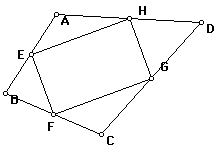We have proved before that this midpoint figure is a parallelogram. Now let's construct ABCD from the midpoint figure.

Experiment 3B. From midpoints to quadrilateral

If you see the connection between the quadrilateral figure and the triangle midpoint figure, it may not surprise you that there is a way to think about this figure using point reflections.

1. In a new sketch draw points A, B, C and P. Construct P' as the point reflection of P with center A.
2. Then construct P'' as the point reflection of P' with center B. Finally, form P''' as the point reflection of P'' with center C. The point P''' is the transform of P by a triple composition, P''' = H_C H_B H_A (P). What (single) transformation does this triple composition appear to be?
3.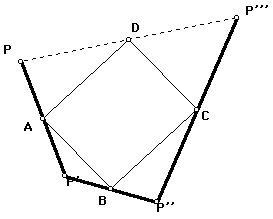4. Investigate in the sketch. Connect P and P''' by a segment and construct its midpoint D. As you drag P around the plane, while leaving A, B, C fixed, does D move?
5. Explain these relationships using the figure. H_B H_A = H_C H_D. From this, H_D = H_C H_B H_A.

Related Problem: Construct a quadrilateral with vertex P given the midpoint parallelogram ABCD. Explain why there is a solution on if ABCD is a parallelogram and only one solution.

We say a shape tessellates the plane, if congruent copies of the shape can be laid down edge to edge as tiles cover a floor. We know some common shapes tile the plane. For example, equilateral triangles tile the plane; also squares or rectangles can be used. In fact general parallelograms tile the plane like this.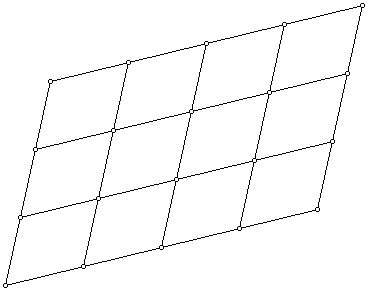Experiment 4A: Sketchpad Construction of Parallelogram Tessellation

1. In a new sketch, begin with a segment AB and mark vector AB. Then use this vector to translate B and its images and images of images to get a row of equally spaced points on line AB. For later use it will be helpful to construct about 8 such points. If he spacing is too wide for the screen, simply drag B closer to A.
2. Next, draw a point C and mark vector AC. Translate all the points previously constructed on AB by this vector and then translate the image and the image of the image to get what is called a "lattice" of points like the vertex points in the parallelogram figure above. Again, try to make the figure about 8 parallelograms across and 8 up.
3. Fill in any missing segments. Your figure may have more parallelograms than the figure above. In principle, the construction could go on all directions to cover the whole plane.
4. SAVE this figure.

Experiment 4B: Hands-on Experiment with General Quadrilateral Tessellations

This shows that special quadrilaterals can tile the plane. But what if we take as a tile a "random" quadrilateral with no special shape. Can we lay down tiles of this shape to cover the plane?

A geometrical fact that may seem surprising is that given any quadrilateral, the plane can be tessellated, or tiled, by congruent copies of that quadrilateral.

Cut out a set of congruent quadrilaterals like this one, or one of your own choosing.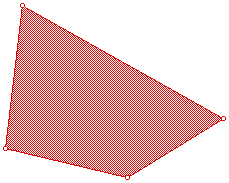Assemble them like a puzzle to cover (tessellate) the plane. Do this! Then note carefully that adjoining shapes are can be moved one onto the other by an isometry. What isometry is this? (Save the figure by taping it to paper or tracing around the shapes onto paper.

Try another example. This time take a shape that is not convex and tile the plane with it. Answer the same question about how neighboring tiles are related by isometries.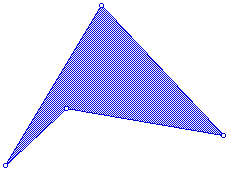In a new sketch, draw a quadrilateral ABCD (no special shape).

Create a tessellation with Sketchpad like the one you did by hand. An important feature of the Sketchpad construction is that if you drag any one of A, B, C or D to change the shape of the original quadrilateral, the shape of ALL the quadrilaterals will change so that all are congruent. And the tiles will still cover the plane. To do this you will need to use transformations. Save this figure!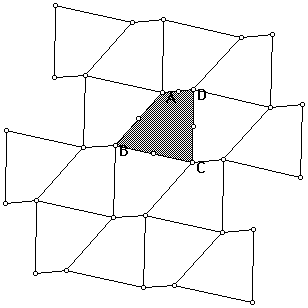Experiment 4C continued: The Midpoint Connection.

Form the midpoint parallelograms of the quadrilaterals and observe how they fit together. How are these parallelograms related to the translations that carry certain quadrilaterals to others? Discuss everything in the light of what you know about composition of point reflections.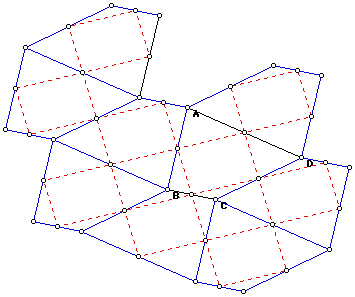When the quadrilaterals are put together to tile the plane, the midpoint parallelograms fit together to give a tiling by parallelograms. The sides of the parallelograms are half the length and direction of the translations that take the tiling to itself.

Experiment 4D: From parallelograms to general quadrilaterals

Return to your sketch with tessellation by parallelograms. Place a point P inside one of the parallelograms with vertices EFGH. Reflect P in each of the vertices E, F, G, and H and connect P to each reflection image point by a segment.

Now each of the images P' of P are also in a parallelogram. Reflect each of these points in the vertices of their parallelograms and each point to its images.

If you keep repeating this constructing and connecting points and their images, you will soon find you have formed a the same figure as in 4C, but this time starting with the parallelograms rather than with the general quadrilateral!

Question: If you imagine the quadrilateral tessellation pattern in 4D or 4C continuing on over the whole plane, what are the symmetries of this infinite figure?